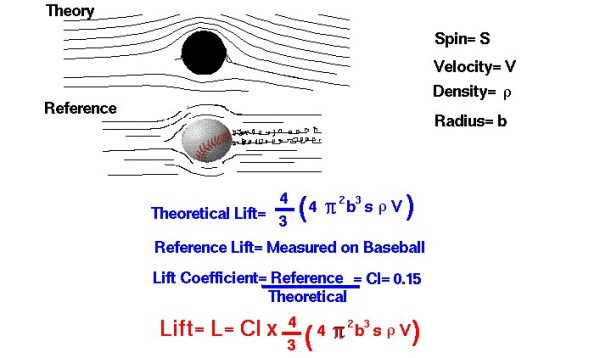# Lift of a BaseballAs an object moves through the air, the air is disturbed by the object. The disturbance generates an aerodynamic force on the object. Since a force is a vector quantity, having both a magnitude and a direction, we can split the aerodynamic force into two components. Drag acts in the direction of travel of the object. Lift acts perpendicular to the direction of travel. Many different objects are capable of generating a lift force. In particular, a spinning ball generates a force that is perpendicular to both the flow direction and to the axis of rotation. The lift force generated by a spinning baseball is the origin of the curve ball thrown by big league pitchers. For a curve ball, the direction of the lift force is used to move the ball parallel to the surface of the earth, from side to side. The lift force on a baseball is too small to overcome the weight of the ball. On this page we will discuss some of the details of the lift of a baseball.

### Aerodynamic Force of a Baseball

The details of how a moving baseball creates lift are fairly complex. If we move with an object through the air, the object appears to stand still and the air moves past the object at the speed of the object. Next to the surface of the object, the molecules of the air stick to the surface, as discussed in the properties of air slide. This thin layer of molecules entrains or pulls the surrounding flow of air. For a spinning ball the external flow is pulled in the direction of the spin. If the ball is not translating, we have a spinning, vortex-like flow set up around the spinning ball, neglecting three-dimensional and viscous effects in the outer flow. If the ball is translating through the air at some velocity, then on one side of the ball the entrained flow opposes the free stream flow, while on the other side of the ball, the entrained and free stream flows are in the same direction. Adding the components of velocity for the entrained flow to the free stream flow, on one side of the ball the net velocity is less than free stream; while on the other, the net velocity is greater than free stream. The flow is then turned by the spinning ball, and a force is generated. Because of the change to the velocity field, the pressure field is also altered around the ball. The magnitude of the force can be computed by integrating the surface pressure times the area around the ball. The direction of the force is perpendicular (at a right angle) to the flow direction and to the axis of rotation. On another page we develop the mathematical equation for the ideal theoretical lift of a spinning, smooth ball.

L = (4 * pi^2 * b^3 * s * rho * V) * 4 / 3

where b is the radius of the ball, s is the spin of the ball, rho is the density of the air, V is the velocity of the ball, and pi is the standard 3.1415…

The equation given above describes the theoretical lift force generated on a smooth, rotating ball. The ideal streamlines around a smooth ball are shown on the figure at the top left, marked “Theory”. But in reality, the flow around a spinning baseball is very complex and looks more like the “Reference” flow shown on the figure. Viscosity generates a boundary layer on the ball and the stitches used to hold the covering of the ball together stick up out of the boundary layer and disturb both the boundary layer and free stream flow. On the ball, the boundary layer transitions to turbulent flow which affects the amount of aerodynamic force. The stitches are not symmetrically distributed around the ball. So the real flow around the ball is separated, unsteady, and not uniform.

### Lift Coefficient of a Baseball

We can use the theoretical lift as a first order, or preliminary, estimate of the lift on a baseball. But to account for these real-world effects that we have neglected in our ideal flow model, we define a lift coefficient. The lift coefficient is an experimentally determined factor that is multiplied times the ideal lift value to produce a real lift value. The ideal simplified model tells us the relative importance of the factors that affect the lift force on the baseball, while all of the complex factors are modeled by the lift coefficient. Using experimental data for the movement of a big league curve ball, we have determined the value of the coefficient to be .15.

The figure on this web page was created by Elizabeth Morton, of Magnificat High School, during a “shadowing” experience at NASA Glenn during May of 2007.